Home

#### The Six Pillars of Calculus

A picture is worth 1000 words

#### Trigonometry Review

The basic trig functions
Basic trig identities
The unit circle
Addition of angles, double and half angle formulas
The law of sines and the law of cosines
Graphs of Trig Functions

#### Exponential Functions

Exponentials with positive integer exponents
Fractional and negative powers
The function $f(x)=a^x$ and its graph
Exponential growth and decay

#### Logarithms and Inverse functions

Inverse Functions
How to find a formula for an inverse function
Logarithms as Inverse Exponentials
Inverse Trig Functions

#### Intro to Limits

Overview
Definition
One-sided Limits
When limits don't exist
Infinite Limits
Summary

#### Limit Laws and Computations

Limit Laws
Intuitive idea of why these laws work
Two limit theorems
How to algebraically manipulate a 0/0?
Indeterminate forms involving fractions
Limits with Absolute Values
Limits involving indeterminate forms with square roots
Limits of Piece-wise Functions
The Squeeze Theorem

#### Continuity and the Intermediate Value Theorem

Definition of continuity
Continuity and piece-wise functions
Continuity properties
Types of discontinuities
The Intermediate Value Theorem
Summary of using continuity to evaluate limits

#### Limits at Infinity

Limits at infinity and horizontal asymptotes
Limits at infinity of rational functions
Which functions grow the fastest?
Vertical asymptotes (Redux)
Summary and selected graphs

#### Rates of Change

Average velocity
Instantaneous velocity
Computing an instantaneous rate of change of any function
The equation of a tangent line
The Derivative of a Function at a Point

#### The Derivative Function

The derivative function
Sketching the graph of $f'$
Differentiability
Notation and higher-order derivatives

#### Basic Differentiation Rules

The Power Rule and other basic rules
The derivative of $e^x$

#### Product and Quotient Rules

The Product Rule
The Quotient Rule

#### Derivatives of Trig Functions

Necessary Limits
Derivatives of Sine and Cosine
Derivatives of Tangent, Cotangent, Secant, and Cosecant
Summary

#### The Chain Rule

Two Forms of the Chain Rule
Version 1
Version 2
Why does it work?
A hybrid chain rule

#### Implicit Differentiation

Introduction
Examples
Derivatives of Inverse Trigs via Implicit Differentiation
A Summary

#### Derivatives of Logs

Formulas and Examples
Logarithmic Differentiation

In Physics
In Economics
In Biology

#### Related Rates

Overview
How to tackle the problems

#### Linear Approximation and Differentials

Overview
Examples
An example with negative $dx$

#### Differentiation Review

How to take derivatives
Basic Building Blocks
Product and Quotient Rules
The Chain Rule
Combining Rules
Implicit Differentiation
Logarithmic Differentiation
Conclusions and Tidbits

#### Absolute and Local Extrema

Definitions
The Extreme Value Theorem
Critical Numbers
Steps to Find Absolute Extrema

#### The Mean Value and other Theorems

Rolle's Theorems
The Mean Value Theorem
Finding $c$

#### $f$ vs. $f'$

Increasing/Decreasing Test and Critical Numbers
Process for finding intervals of increase/decrease
The First Derivative Test
Concavity
Concavity, Points of Inflection, and the Second Derivative Test
The Second Derivative Test
Visual Wrap-up

#### Indeterminate Forms and L'Hospital's Rule

What does $\frac{0}{0}$ equal?
Examples
Indeterminate Differences
Indeterminate Powers
Three Versions of L'Hospital's Rule
Proofs

Strategies
Another Example

#### Newton's Method

The Idea of Newton's Method
An Example
Solving Transcendental Equations
When NM doesn't work

#### Anti-derivatives

Antiderivatives
Common antiderivatives
Initial value problems
Antiderivatives are not Integrals

#### The Area under a curve

The Area Problem and Examples
Riemann Sum Notation
Summary

#### Definite Integrals

Definition of the Integral
Properties of Definite Integrals
What is integration good for?
More Applications of Integrals

#### The Fundamental Theorem of Calculus

Three Different Concepts
The Fundamental Theorem of Calculus (Part 2)
The Fundamental Theorem of Calculus (Part 1)
More FTC 1

#### The Indefinite Integral and the Net Change

Indefinite Integrals and Anti-derivatives
A Table of Common Anti-derivatives
The Net Change Theorem
The NCT and Public Policy

#### Substitution

Substitution for Indefinite Integrals
Examples to Try
Revised Table of Integrals
Substitution for Definite Integrals
Examples

#### Area Between Curves

Computation Using Integration
To Compute a Bulk Quantity
The Area Between Two Curves
Horizontal Slicing
Summary

#### Volumes

Slicing and Dicing Solids
Solids of Revolution 1: Disks
Solids of Revolution 2: Washers
More Practice

### Slicing and Dicing Solids

Main idea:  To find the volume of a solid, we slice the solid into thin pieces and add up the volumes of the pieces.

When we slice a solid, we get cross-sections of the solid.  For example, when we slice a solid cylinder, we see that we can get cross-sections that are rectangles or circles, depending upon how we slice.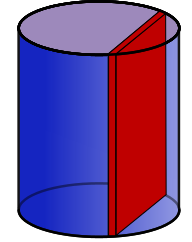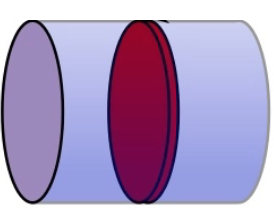http://calculus.seas.upenn.edu/

In general, we do not have nice solids like a cylinder, but we can slice any solid.  We put our solid on axes, so that we can coordinatize our slices, as in the diagrams below.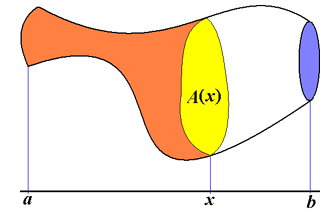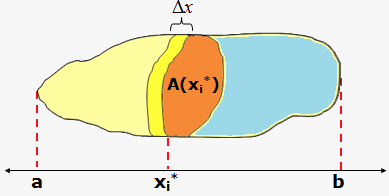http://www2.bc.cc.ca.us/                                                        http://www.emathhelp.net

We slice the solid into these cross-sectional pieces, then add up the volumes of each of the pieces, to get the volume of the solid.  If a slice has cross-sectional area $A(x)$ and thickness $\Delta x$ at each particular $x$-value, then the slice has approximate volume $A(x) \Delta x$. Adding up the volumes of the slices and taking a limit as we slice finer and finer, we get

 $$\hbox{Volume} = \int_a^b A(x)\, dx$$

where $a$ and $b$ are the $x$-values of the leftmost and rightmost slices.

Of course, we could also slice horizontally,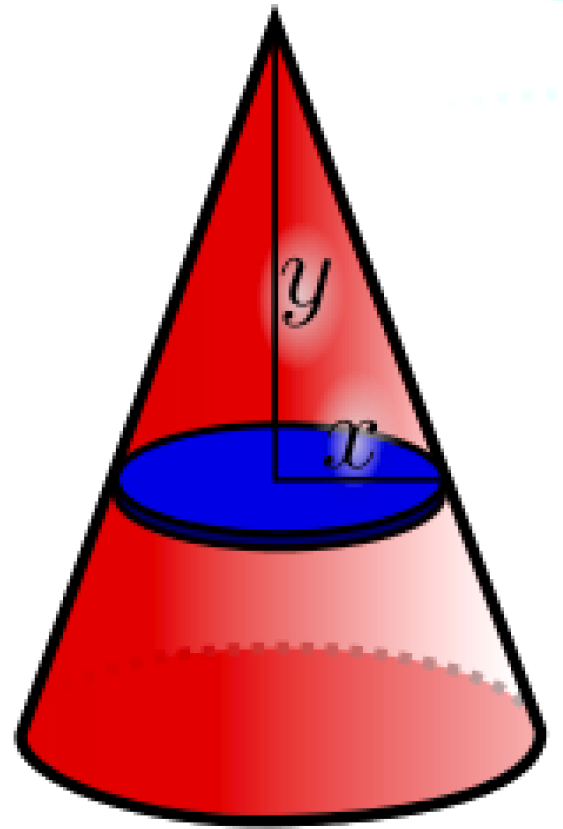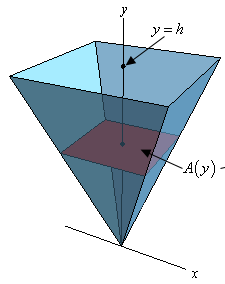http://calculus.seas.upenn.edu/                         http://tutorial.math.lamar.edu/
in which case we would find the volume to be $\int_c^d A(y)\,dy$, where $c$ is the smallest $y$-value, and $d$ is the largest ($c=0,d=h$ in the second diagram above).

The video will make these ideas more concrete.